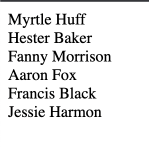# const - JS | lectureNumbers, Dates, Intl & Timers

The Remainder Operator

One operator that I didn't mention in the last lecture is the Remainder Operator. And the reason for that is that the remainder operator has some special use cases, so it deserves a lecture on its own.

So what is the remainder operator? Well, as the name says, the remainder operator returns the remainder of a division. Let's see how that works.

Let's say we want to know what the remainder of 5 divided by 2 is.

script.js
``console.log(5 % 2); // 1``

Why do we have this result 🤔? Well because if we divide 5 by 2, we get 2.5.

script.js
``console.log(5 / 2); // 2.5``

But if we took only the integer part of that number, we would get 2. Because 2 * 2 is 4, but then, there will be a remainder of 1. Because 5 - 4 is 1. So we can say that 5 is 2 * 2 + 1.

script.js
``````console.log(5 / 2); // 5 = 2 * 2 + 1

// Another example
console.log(8 % 3); // 2
console.log(8 / 3); // 8 = 3 * 2 + 2``````

Next, in programming, we often use the remainder operator to check if a number is even or odd. A number is even if the remainder of the division by 2 is 0. So let's check that.

script.js
``console.log(6 % 2); // 0``

We can see that the remainder of 6 divided by 2 is 0. And that's because, dividing 6 by 2 is an integer number, so it's exactly 3.

script.js
``````console.log(6 / 2); // 3
// 6 = 2 * 3``````

But on the othe hand, an odd number, for example 7, will have a remainder of 1. That's because, 3 * 2 is 6, and then plus 1, which is the remainder, we get 7.

script.js
``````console.log(7 % 2); // 1
console.log(7 / 2); // 7 = 3 * 2 + 1``````

We can now use this knowledge to write a function that checks if a number is even or odd.

script.js
``````const isEven = (num) => num % 2 === 0;

console.log(isEven(2)); // true
console.log(isEven(23)); // false
console.log(isEven(100)); // true``````

Let's do something fun with this. Consider the below HTML code.

``````<div class="names">
<div class="name_row">Myrtle Huff</div>
<div class="name_row">Hester Baker</div>
<div class="name_row">Fanny Morrison</div>
<div class="name_row">Aaron Fox</div>
<div class="name_row">Francis Black</div>
<div class="name_row">Jessie Harmon</div>
</div>``````Above, we have a list of names. Let's select all these names and we will change the background color of each name based on a certain condition.

script.js
``const names = document.querySelectorAll('.name_row');``

Remember that the `querySelectorAll` method returns a `NodeList`. So we need to convert it to an array. And for that, we can either use the `Array.from` method or the spread operator. I will use the spread operator.

script.js
``const names = [...document.querySelectorAll('.name_row')];``

Now on the names array, we can use the `forEach` method to loop through each name.

script.js
``````names.forEach((name, index) => {
// do something
});``````

Let's now say that I want to change the background color of every second row of the names. So all I need to do is to check if the index of the name is even.

script.js
``````names.forEach((name, index) => {
if (isEven(index)) {
name.style.backgroundColor = '#a0ce8f';
}
});``````So this is how we use the remainder operator, yet another one in your toolbox. And as always, if you have any questions, feel free to ask them in the comments below, or you can also have a look at the documentation on MDN.